Treasury rates have a tremendous impact on financial assets through their influence on the cost of borrowing. Therefore, understanding the relationship between treasury rates and financial assets is of primary importance. We found that while treasury rates are positively related to financial assets such as oil and US dollar, they are negatively related to S&P500 and gold. We also found that such relationships become unstable and unpredictable when treasury rates are at very low levels.

## Historical Performance of Treasury Rates and All Major Financial Assets

Treasury rates are important signals of the economy and investors’ expectations. Since summer 2019, the Fed began to cut the rates to protect America from the trade wars and the slowing global economy. In March 2020, the Fed further cut the rate to bolster the markets through the pandemic. The treasury rates are now at historical low levels. Figure 1 shows the historical level of treasury rates and the inflation, showing how treasury rates kept declining since 1981.

Figure 2 shows the Two-year Treasury Constant Maturity Rate and YoY Inflation rate. As the treasury rate embeds the inflation expectation, which is influenced by the real inflation rate, we could observe a high correlation between the treasury rate and the inflation, which is 0.73 during our study’s time period. Figure 3 shows the value of \$1 investment in S&P 500, Oil, Gold and US Dollar. During our study’s timeframe, an investment in equities brings the highest return, followed by gold.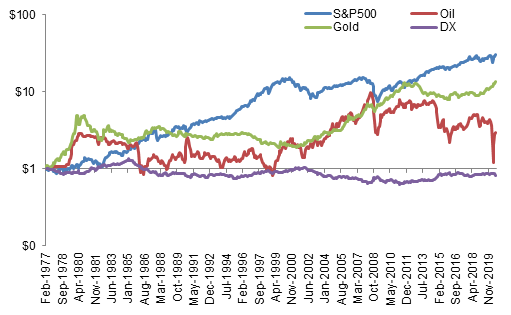Figure 3 Value of \$1 investment in S&P 500, Oil, Gold and US Dollar (log scale)

## Relationship between Treasury Rates and Major Financial Assets

To analyze the relationship between treasury rates and financial assets, we chose the 2-year and 10-year Treasury rates as the representative variables for our analyses since they better represent the sector as they are the most liquid and highly traded among all the maturities.

### S&P 500 (SPY)

The equity market is sensitive to treasury rates as it competes with fixed income market for capitals of investors. Also, lower rates allow companies to borrow money at a lower cost to expand their business. Usually a low treasury rate would drive the price of equity up. As long-term investments are more risky than short-term ones in general, investors will ask for higher returns for long-term bonds. Therefore, the ten-year treasury rate is higher than the rate of two-year treasury on average. Figure 4 shows the annual return of S&P 500 against the spread between two-year and ten-year treasury rates. When the spread widen, S&P 500 return tends to increase, such as 1981-1984 and 1990-1994. When the spread narrows, indicating investors are looking for a safe investment, equity market tends to plunge because of its high volatility and risks. This is what we observed during the period 1998-2003 in Figure 4. Following a consistent narrowing of spread through 1992 to 2000, the S&P 500 suffered a tremendous drop.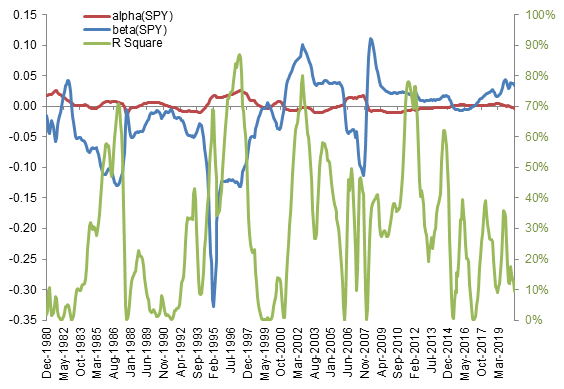Figure 5 Rolling 3-year Regression of S&P 500 Annual Return on 12-month Changes of 2-Year Treasury Rate

Figure 5 shows the 3-year rolling regression of S&P 500 annual return on 12-month changes of two-year treasury rate. For most of the time, the equity market is negatively related to the treasury rate, with a higher explanatory power of the regression model. However, such relationship is not constant. As the treasury rates are at historical low levels, it becomes more difficult to detect the relationship between the equity market and treasury rates.

Figure 8 shows the 12-month changes of the 2-year Treasury Rate vs. the S&P500 annual return. As indicating in the regression models (Table 1), the annual return of S&P500 is impacted by the 2-year treasury rate, which could also be detected from Figure 8 that SPY moves in tandem with the lagged delta of 2Y treasury rates..

### Oil

We conducted same analyses to oil. Figure 9 shows the rolling regression of oil annual return on 12-month changes of the two-year treasury rate. Unlike betas of equity, betas of the rolling regression of are mainly positive.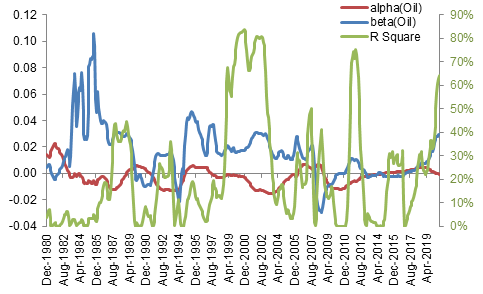Figure 9 Rolling 3 year Regression of Oil Annual Return on 12-month Changes of 2Y Treasury Rate

According to the regression results in Table 2, the movement of oil price tends to follow the changes of treasury rate not the other way around, as R square increased from 0.31% to 1.18% while changing the dependent variable from delta of the treasury rate to annual return of oil. Figure 12 also shows that oil price and lagged treasury rates usually move in tandem.

Gold, as one of the safest investment, reflects the economic conditions and investors’ expectations. Usually the price of gold would increase when markets are not doing well and investors are rushing to safe investments.  However, sometimes, rising rates in short-term would put pressures on gold price as investors fly to US bonds. Figure 13 shows the results of rolling regressions. The relationship between gold and two-year treasury rate changes over time.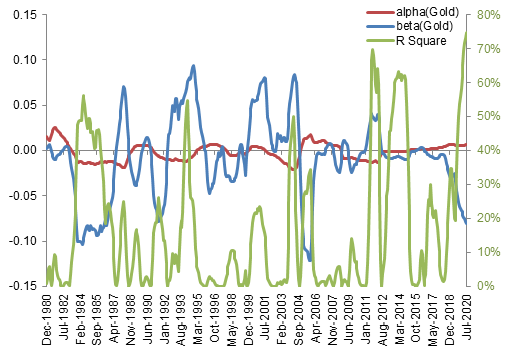Figure 13 Rolling 3 year Regression of Gold Annual Return on 12-month Changes of 2Y Treasury Rate

Unlike SPY and Oil, the regression model with Annual Return of Gold as independent variable has a higher R square, suggesting that gold could be an indicator of future changes of two-year treasury rates (Table 3). Figure 15 corresponds to the1st regression in Table 3, where gold and lagged 2Y treasury rate are more related to each other.

### US Dollar (DX)

As treasury rates directly impact the demand of a currency, treasury rates will impact the exchange rate. Figure 17 shows the results of rolling regression. The average of betas is 0.018, indicating the exchange rate is positively related to the treasury rates.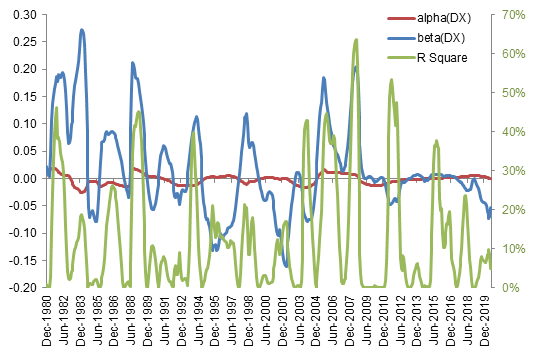Figure 17 Rolling 3 year Regression of DX Annual Return on 12-month Changes of 2Y Treasury Rate

Table 4 shows the regression results. The regression models didn’t show a strong difference between each other, indicating that the exchange rate moves quickly in response to the changes of treasury rates. Such relationship could also be spotted from Figure 18-20, as there is no strong difference among those three time series plots.